# Introduction

In the adaptation level experiment participants had to assess weights of the objects placed in their hands by using a verbal scale: very very light, very light, light, medium light, medium, medium heavy, heavy, very heavy and very very heavy. The task was to assess the weight of an object that was placed on the palm of their hand. To standardize the procedure the participants had to place the elbow on the desk, extend the palm and assess the weight of the object after it was placed on their palm by slight up and down movements of their arm. During the experiment participants were blinded by using non-transparent fabric. In total there were 15 objects of the same shape and size but different mass (photo film canisters filled with metallic balls). Objects were grouped into three sets:

• light set: 45 g, 55 g, 65 g, 75 g, 85 g (weights 1 to 5),
• medium set: 95 g, 105 g, 115 g, 125 g, 135 g (weights 6 to 10),
• heavy set: 145 g, 155 g, 165 g, 175 g, 185 g (weights 11 to 15).

The experimenter sequentially placed weights in the palm of the participant and recorded the trial index, the weight of the object and participant’s response. The participants were divided into two groups, in group 1 the participants first assessed the weights of the light set in ten rounds within which all five weights were weighted in a random order. After completing the 10 rounds with the light set, the experimenter switched to the medium set, without any announcement or break. The participant then weighted the medium set across another 10 rounds of weighting the five weights in a random order within each round. In group 2 the overall procedure was the same, the only difference being that they started with the 10 rounds of the heavy set and then performed another 10 rounds of weighting of the medium set. Importantly, the weights within each set were given in random order and the experimenter switched between sets seamlessly without any break or other indication to the participant.

We will use the bayes4psy package to show that the two groups provide different assessment of the weights in the second part of the experiment even though both groups are responding to weights from the same (medium) set. The difference is very pronounced at first but then fades away with subsequent assessments of medium weights. This is congruent with the hypothesis that each group formed a different adaptation level during the initial phase of the task, the formed adaptation level then determined the perceptual experience of the same set of weights at the beginning of the second part of the task.

We will conduct the analysis by using the hierarchical linear model. First we have to construct fits for the second part of the experiment for each group independently. The code below loads and prepares the data, just like in the previous example, subject indexes have to be mapped to a [1, n] interval. We will use to ggplot2 package to fine-tune graph axes and properly annotate graphs returned by the bayes4psy package.

# libs
library(bayes4psy)
library(dplyr)
library(ggplot2)

# separate groups and parts
group1_part2 <- data %>% filter(group == 1 & part == 2)
group2_part2 <- data %>% filter(group == 2 & part == 2)

Once the data is prepared we can fit the Bayesian models, the input data comes in the form of three vectors, $$x$$ stores indexes of the measurements, $$y$$ subject’s responses and $$s$$ indexes of subjects. Note here that, due to vignette limitations, all fits are built using only one chain, using more chains in parallel is usually more efficient. Also to increase the building speed of vignettes we greatly reduced the amount of iterations, use an appropriate amount of iterations when executing actual analyses!

# fit
fit1 <- b_linear(x=group1_part2$sequence, y=group1_part2$response,
s=group1_part2$subject, iter=200, warmup=100, chains=1) fit2 <- b_linear(x=group2_part2$sequence,
y=group2_part2$response, s=group2_part2$subject,
iter=200, warmup=100, chains=1)

The fitting process is always followed by the quality analysis.

# trace plots
plot_trace(fit1)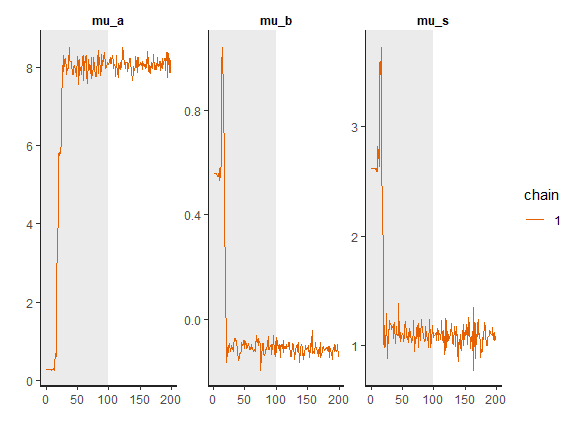plot_trace(fit2)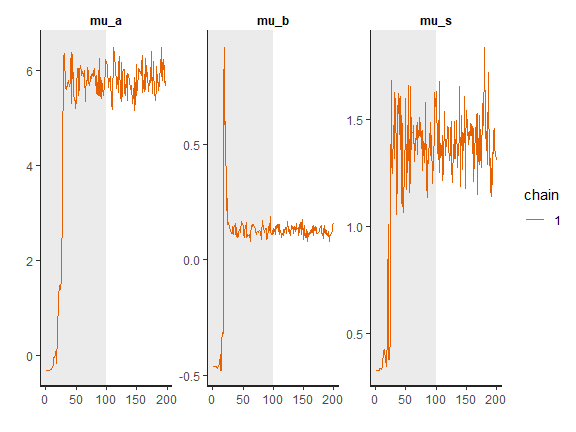# the commands below are commented out for the sake of brevity
#print(fit1)
#print(fit2)

# visual inspection
plot(fit1)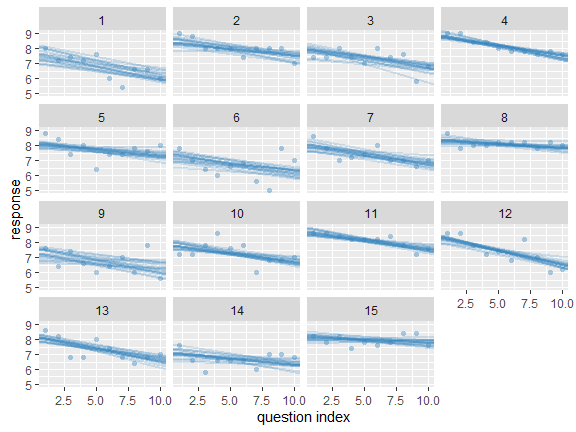plot(fit2)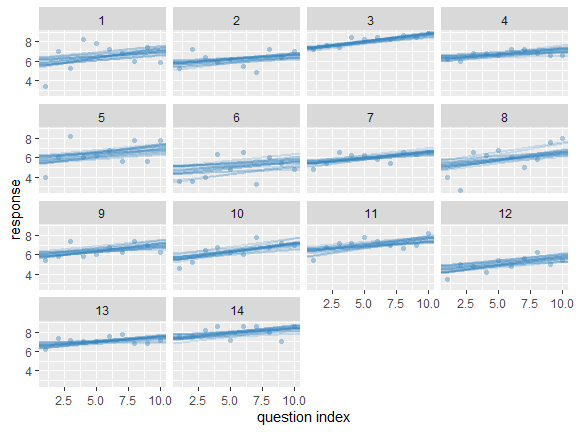The trace plot showed no MCMC related issues, effective sample sizes of parameters relevant for our analysis ($$\mu_a$$, $$\mu_b$$ and $$\mu_s$$) are large enough. Since the visual inspection of the fit also looks good we can continue with our analysis. To get a quick description of fits we can take a look at the summary statistics of model’s parameters.

summary(fit1)
## intercept (alpha):   8.07 +/- 0.01648, 95% HDI: [7.73, 8.37]
## slope (beta):        -0.12 +/- 0.00195, 95% HDI: [-0.15, -0.09]
## sigma:           1.08 +/- 0.00915, 95% HDI: [0.88, 1.24]
summary(fit2)
## intercept (alpha):   5.82 +/- 0.03564, 95% HDI: [5.31, 6.48]
## slope (beta):        0.12 +/- 0.00250, 95% HDI: [0.09, 0.16]
## sigma:           1.40 +/- 0.01302, 95% HDI: [1.14, 1.66]

Values of intercept suggest that our initial hypothesis about adaptation level is true. Subject’s that weighted lighter object in the first part of the experiment (fit1) find medium objects at the beginning of experiment’s second part heavier than subjects that weighted heavier objects in the first part (fit2). We can confirm this assumption by using functions that perform a more detailed analysis (e.g. compare_means and plot_means_difference, see the outputs below).

comparison_results <- compare_means(fit1, fit2=fit2)
## ---------- Intercept ----------
## Probabilities:
##   - Group 1 < Group 2: 0.00 +/- 0.00000
##   - Group 1 > Group 2: 1.00 +/- 0.00000
## 95% HDI:
##   - Group 1 - Group 2: [1.50, 2.96]
##
## ---------- Slope ----------
## Probabilities:
##   - Group 1 < Group 2: 1.00 +/- 0.00000
##   - Group 1 > Group 2: 0.00 +/- 0.00000
## 95% HDI:
##   - Group 1 - Group 2: [-0.30, -0.20]
plot_means_difference(fit1, fit2=fit2, par="intercept")
##
## ---------- Using only the intercept parameter. ----------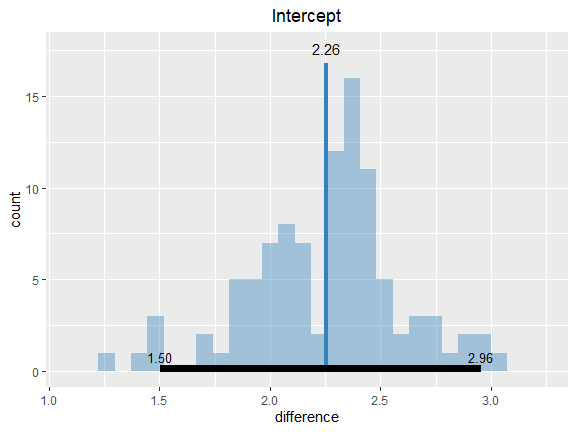The fact that the slope for the first group is very likely to be negative (the whole 95% HDI lies below 0) and positive for the second group (the whole 95% HDI lies above 0) suggests that the adaptation level phenomenon fades away with time. We can visualize this by plotting means and distributions underlying both fits. The plotting functions in the bayes4psy package return regular ggplot2 plot objects, so we can use the same techniques to annotate or change the look and feel of graphs as we would with the usual ggplot2 visualizations.

plot_distributions(fit1, fit2) +
labs(title="Part II", x="measurement number", y="") +
theme(legend.position="none") +
scale_x_continuous(limits=c(1, 10), breaks=seq(1:10)) +
ylim(0, 10)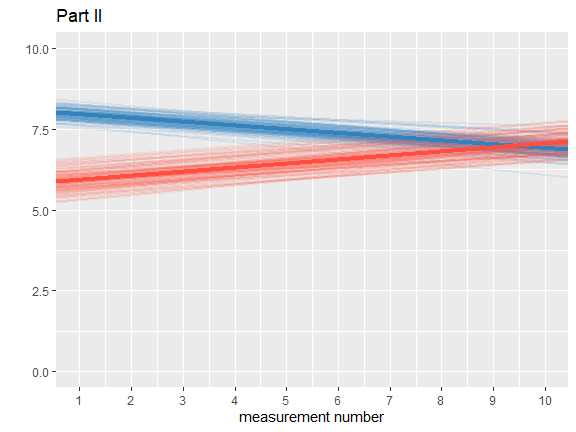Based on the analysis above, the hypothesis that each group formed a different adaptation level during the initial phase of the task seems to be true. Group that switches from heavy to medium weights assesses weights as lighter than they really are while for the group that switches from light to medium the weights appear heavier. With time these adaptation levels fade away and assessments converge to similar estimates of weight.